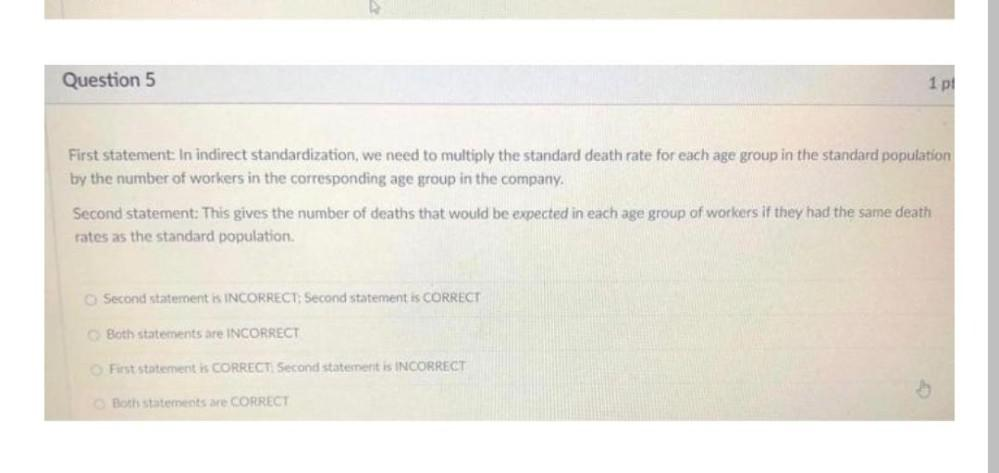Question:

# Question 5 1 pt First statement In indirect standardization, we need to multiply the standard death rate for each age group in tQuestion 5 1 pt First statement In indirect standardization, we need to multiply the standard death rate for each age group in the standard population by the number of workers in the corresponding age group in the company, Second statement: This gives the number of deaths that would be expected in each age group of workers if they had the same death rates as the standard population Second statement is INCORRECT: Second statement is CORRECT Both statements are INCORRECT First statement is CORRECT Second statement is INCORRECT Both statements are CORRECT